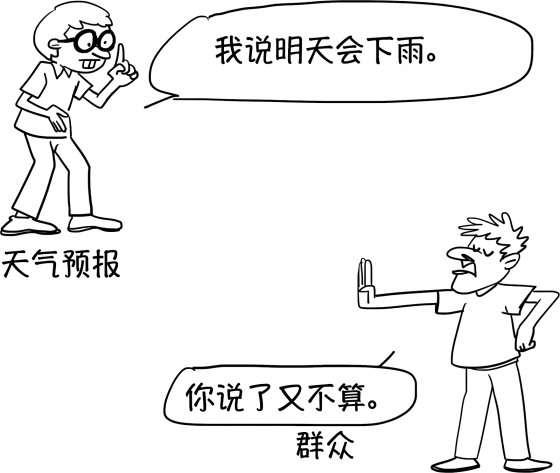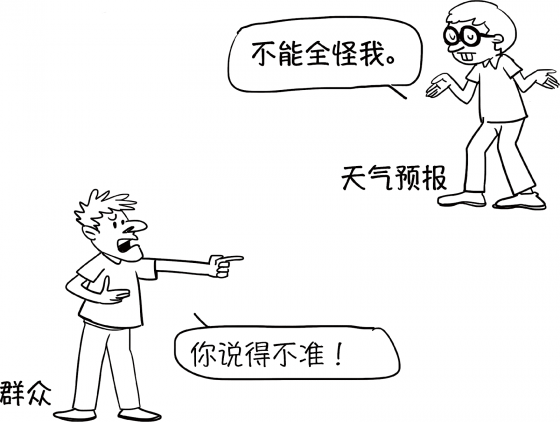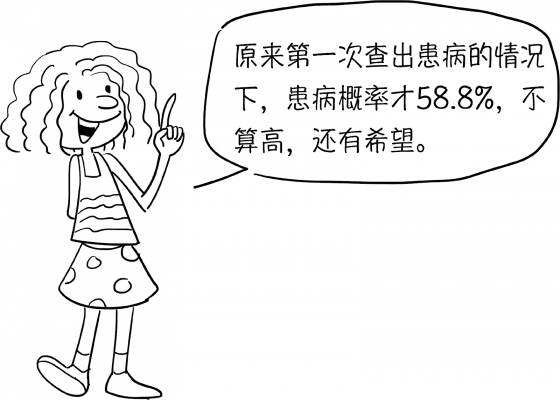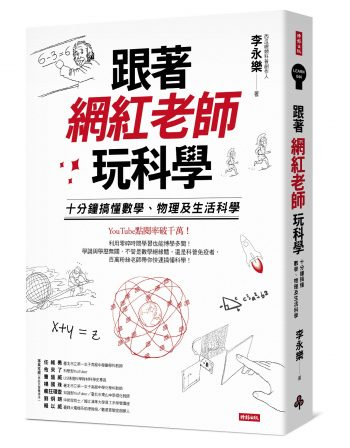# 天氣預報到底是不是在騙人？我整個就不爽了！從生活案例看條件機率——《跟著網紅老師玩科學》

2019/08/23 | | 標籤：## 你哭著對我說，天氣預報裡都是騙人的

 下雨 不下雨 預報下雨 40% × 90% = 36% 60% × 10% = 6% 預報不下雨 40% × 10% = 4% 60% × 90% = 54%## 檢查報告說我中獎了，我就真的生病了嗎？

 患病 健康 檢測患病 $$\frac{1}{7000} \times \frac{9999}{10000}$$$$= \frac{9999}{70000000}$$ $$\frac{6999}{7000} \times \frac{1}{10000}$$$$= \frac{6999}{70000000}$$ 檢測健康 $$\frac{1}{7000} \times \frac{1}{10000}$$$$= \frac{1}{70000000}$$ $$\frac{6999}{7000} \times \frac{9999}{10000}$$$$= \frac{69983001}{70000000}$$患病 健康 檢測患病 58.8% × $$\frac{9999}{10000}$$= 58.794% 41.2% × $$\frac{1}{10000}$$= 0.004% 檢測健康 58.8% × $$\frac{1}{10000}$$= 0.006% 41.2% × $$\frac{9999}{10000}$$= 41.196%

## 日常生活超有感──貝式定理$$P(B_1)P(A_1|B_1)+P(B_2)P(A_2|B_2)+\cdots+P(B_n)P(A_n|B_n)$$

$$P(B_i|A)$$ $$=\frac{P(B_i)P(A|B_i)}{P(B_1)P(A|B_1)+P(B_2)P(A|B_2)+\cdots+P(B_n)P(A|B_n)}$$——本文摘自《跟著網紅老師玩科學》，2019 年 4 月，時報出版

【時時科科．2020】桌曆＋線裝筆記本預購最後倒數！🚀 泛科學院獨家線上新課募資 🚀 限量55折預購

【好好說話，做自己的口才教練！10堂一生受用的口語表達課】「上台說話報告時腦袋一片空白嗎？與人對談尷尬癌就發作？如何清楚表達自己想說的話？怎麼說話才能抓住人心讓人印象深刻呢？」泛科學院與榮恩同樂會共同合作，從表達的心法到語言聲韻的技巧掌握，讓你找到自信，在家就可練出好口才！

## 關於作者